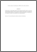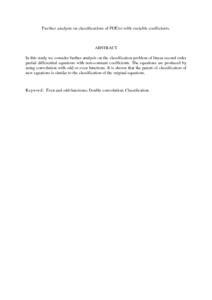Simple Search:

# Further analysis on classifications of PDE(s) with variable coefficients.

## Citation

Kilicman, Adem and H., Eltayeb and Radzhabovich, Ashurov Ravshan (2010) Further analysis on classifications of PDE(s) with variable coefficients. Applied Mathematics Letters, 23 (9). pp. 966-970. ISSN 0893-9659

## Abstract / Synopsis

In this study we consider further analysis on the classification problem of linear second order partial differential equations with non-constant coefficients. The equations are produced by using convolution with odd or even functions. It is shown that the patent of classification of new equations is similar to the classification of the original equations.Preview
PDF (Abstract)
Further analysis on classifications of PDE.pdfView Item